## Saturday, April 25, 2015

### Some updates to plotting functions & a new phytools version

I just posted a new version of phytools. The main updates of this version is that a number of phytools functions (`plotSimmap`, `plotTree`, `fancyTree`, `contMap`, `densityMap`, and `phenogram`) now have the option of hold the output to the graphical device using `dev.hold` before plotting. Depending on your operating system, this may have the effect of delaying the plot from starting to appear - but then all the elements should appear all at once. This is really only important for computationally intensive methods and for very large trees. (It can also be turned off if the user wants to see the plot appear gradually. It takes just as long anyway, so sometimes it is nice to have something to look at!)

Obviously, it is not possible in the blog to illustrate the effect of turning this option on - all you get to see is the final plotted object anyway - but I thought this would be a nice moment to remind me readers (all 2 or 3 of you) what some of the above functions can do.

1. `plotSimmap`

Obviously, `plotSimmap` plots stochastic character mapped trees, or other trees that have been stored in the type of object created by `read.simmap` & `make.simmap` (among the other functions that work with this type of object). Here is a demo of what this kind of plot looks like:

``````library(phytools)
data(anoletree)
plotSimmap(anoletree,type="fan",fsize=0.7,ftype="i")
``````
``````## no colors provided. using the following legend:
##        CG        GB        TC        TG        Tr        Tw
##   "black"     "red"  "green3"    "blue"    "cyan" "magenta"
``````
``````## setEnv=TRUE for this type is experimental. please be patient with bugs
``````
``````ss<-sort(unique(getStates(anoletree)))
x=-9.3,y=-6)
``````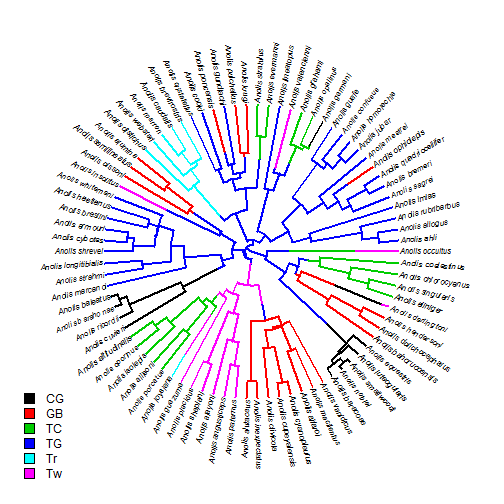2. `plotTree`

This is just a wrapper for `plotSimmap` that plots a tree without a mapped character, but includes all the user options as in `plotSimmap`.

3. `phenogram`

This function plots a projection of the tree into a space defined by time since the root on the horizontal axis and phenotype for a continuously valued trait on the vertical. It can work with user-supplied or reconstructed trait values at internal nodes. Here is what that looks like:

``````## simulate tree & data
tree<-pbtree(n=26,tip.label=LETTERS)
x<-fastBM(tree)
phenogram(tree,x,fsize=0.8)
``````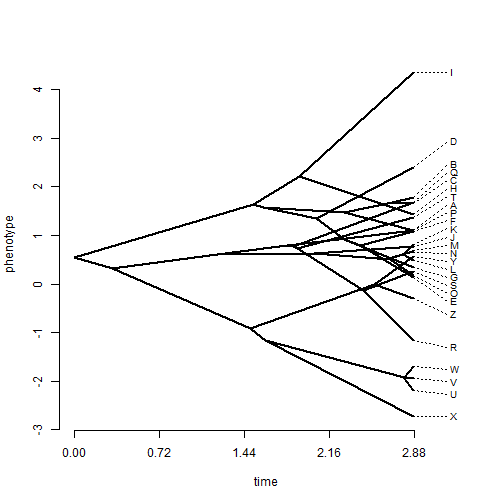In this version I have also updated the default settings to `spread.labels=FALSE` - because these plots are much more aesthetically pleasing (and readable) than the alternative:

``````phenogram(tree,x,fsize=0.8,spread.labels=FALSE)
``````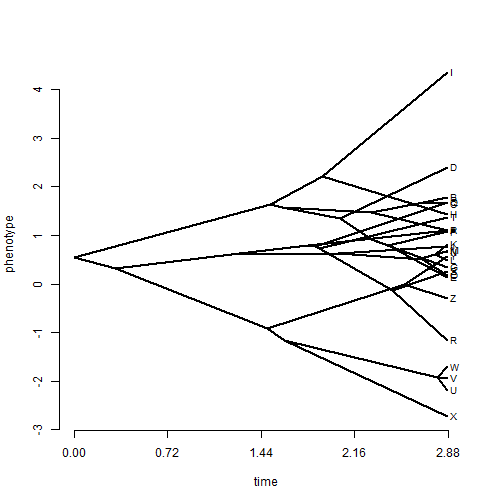4. `fancyTree`

`fancyTree` does a range of idiosyncratic visualizations, but the methods that I have updated are `"phenogram95"` and `"scattergram"`.

`"phenogram95"` uses transparency to plot a 95% (by default) high probability density traitgram. For example:

``````fancyTree(tree,"phenogram95",x=x)
``````
``````## Computing density traitgram...
``````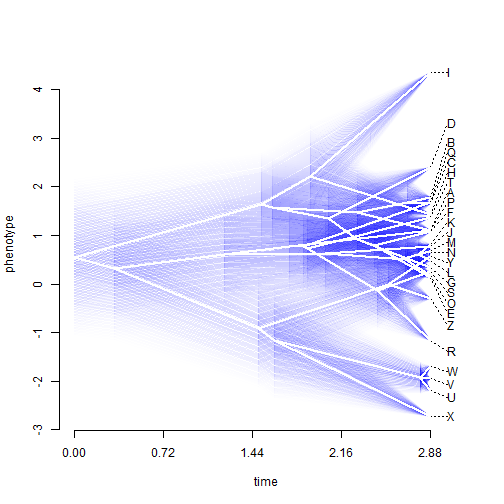This in particular is a method were the plot used to appear very gradually on the graphical device!

`"scattergram"` does a phylogenetic scatterplot matrix with continuous character maps on the tree (see below for more info) on the diagonal, and phylmorphospaces (two-dimensional projections of the tree into morphospace) in off-diagonal matrix positions. Here is a demo once again:

``````## simulate uncorrelated character data
X<-fastBM(tree,nsim=4)
## name columns, just for fun
colnames(X)<-c("size","forelimb","hindlimb","mass")
fancyTree(tree,type="scattergram",X=X)
``````
``````## Computing multidimensional phylogenetic scatterplot matrix...
``````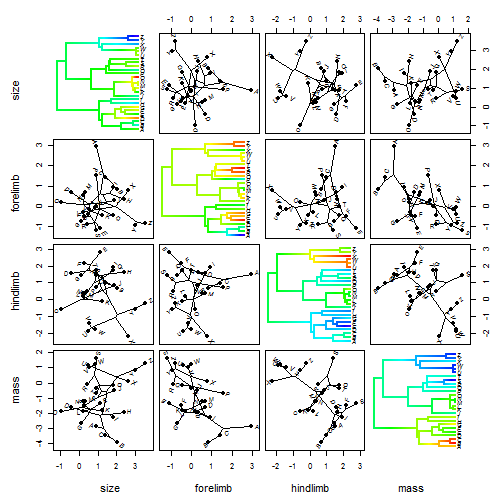5. `densityMap` and `contMap`

Well, we've already seen `contMap` in use. This is just a visualization method in which a continuous character trait value is projected directly onto the edges of the tree. So, for example:

``````tree<-pbtree(n=100)
x<-fastBM(tree)
obj<-contMap(tree,x,fsize=c(0.5,1))
``````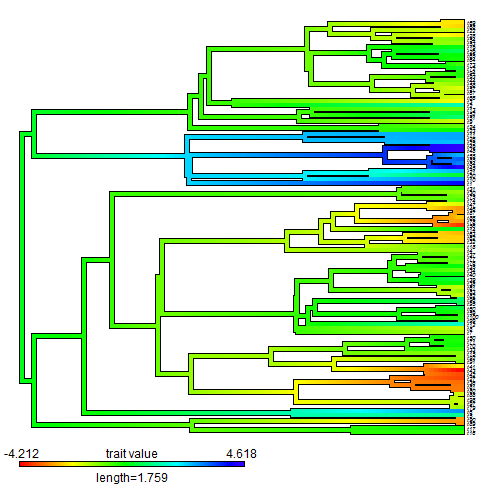`contMap` returns an object of class `"contMap"` which can easily be replotted with different settings, e.g.:

``````plot(obj,type="fan")
``````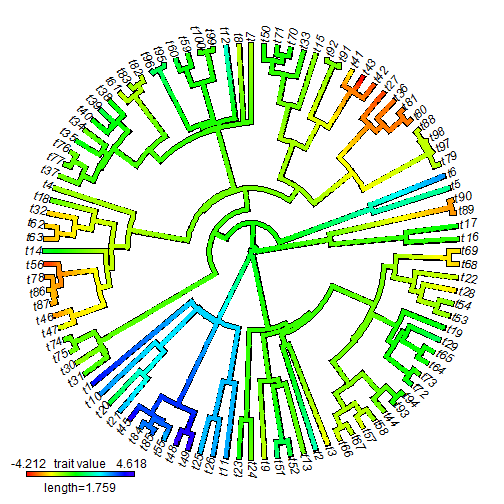A related method is the stochastic character map density method of `densityMap`. I won't get into the details here, but this is just an approach to visualize the posterior probability density along edges and nodes from a statistical ancestral state reconstruction method called stochastic character mapping. Here, once again, is an example using simulated data:

``````tree<-pbtree(n=26,tip.label=LETTERS[26:1],scale=1)
Q<-matrix(c(-1.25,1.25,1.25,-1.25),2,2)
rownames(Q)<-colnames(Q)<-letters[1:2]
tree<-sim.history(tree,Q)
``````
``````## Done simulation(s).
``````
``````cols<-setNames(c("blue","red"),letters[1:2])
plotSimmap(tree,colors=cols,lwd=3) ## simulated true history
``````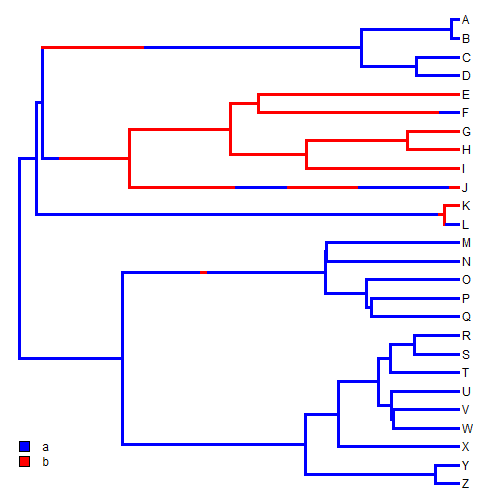``````x<-tree\$states ## states
x
``````
``````##   Z   Y   X   W   V   U   T   S   R   Q   P   O   N   M   L   K   J   I
## "a" "a" "a" "a" "a" "a" "a" "a" "a" "a" "a" "a" "a" "a" "a" "b" "b" "b"
##   H   G   F   E   D   C   B   A
## "b" "b" "a" "b" "a" "a" "a" "a"
``````
``````mtrees<-make.simmap(tree,x,nsim=100)
``````
``````## make.simmap is sampling character histories conditioned on the transition matrix
## Q =
``````
``````##            a          b
## a -0.5107549  0.5107549
## b  0.5107549 -0.5107549
``````
``````## (estimated using likelihood);
## and (mean) root node prior probabilities
## pi =
``````
``````##   a   b
## 0.5 0.5
``````
``````## Done.
``````
``````obj<-densityMap(mtrees)
``````
``````## sorry - this might take a while; please be patient
``````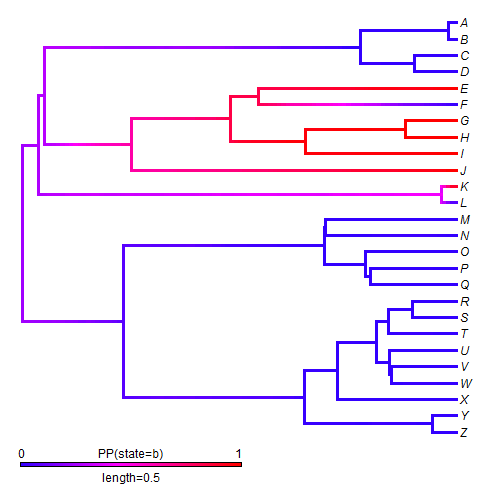``````plot(obj,lwd=5,outline=TRUE,direction="leftwards")
``````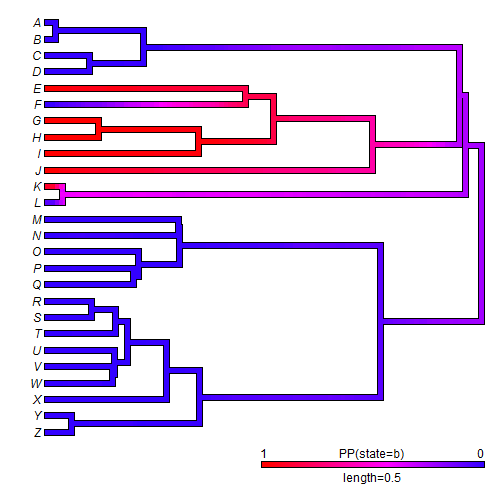OK, that's it. I'm hoping to get a CRAN update out soon, but for now this latest version of phytools can be downloaded from phytools.org or, more specifically, here.